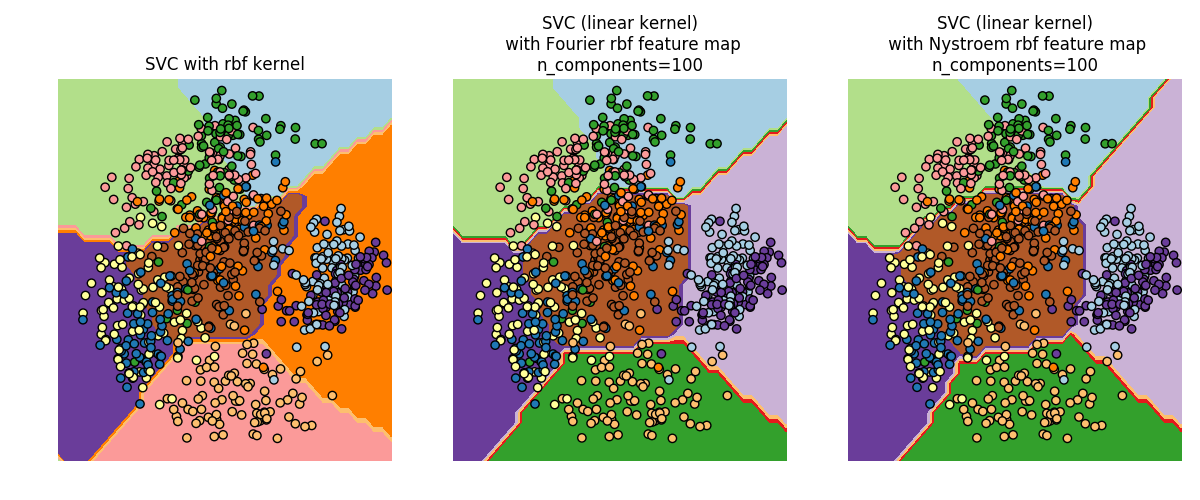# 5.7. 内核近似

kernel trick 相比，近似的进行特征映射更适合在线学习，并能够有效 减少学习大量数据的内存开销。使用标准核技巧的 svm 不能有效的适用到海量数据，但是使用近似内核映射的方法，对于线性 SVM 来说效果可能更好。 而且，使用 SGDClassifier 进行近似的内核映射，使得对海量数据进行非线性学习也成为了可能。

## 5.7.1. 内核近似的 Nystroem 方法

Nystroem 中实现了 Nystroem 方法用于低等级的近似核。它是通过采样 kernel 已经评估好的数据。默认情况下， Nystroem 使用 rbf kernel，但它可以使用任何内核函数和预计算内核矩阵. 使用的样本数量 - 计算的特征维数 - 由参数 n_components 给出.

## 5.7.2. 径向基函数内核

RBFSampler 为径向基函数核构造一个近似映射，又称为 Random Kitchen Sinks [RR2007]. 在应用线性算法（例如线性 SVM ）之前，可以使用此转换来明确建模内核映射:

>>> from sklearn.kernel_approximation import RBFSampler
>>> from sklearn.linear_model import SGDClassifier
>>> X = [[0, 0], [1, 1], [1, 0], [0, 1]]
>>> y = [0, 0, 1, 1]
>>> rbf_feature = RBFSampler(gamma=1, random_state=1)
>>> X_features = rbf_feature.fit_transform(X)
>>> clf = SGDClassifier()
>>> clf.fit(X_features, y)
SGDClassifier(alpha=0.0001, average=False, class_weight=None, epsilon=0.1,
eta0=0.0, fit_intercept=True, l1_ratio=0.15,
learning_rate='optimal', loss='hinge', max_iter=5, n_iter=None,
n_jobs=1, penalty='l2', power_t=0.5, random_state=None,
shuffle=True, tol=None, verbose=0, warm_start=False)
>>> clf.score(X_features, y)
1.0



fit 函数有两个参数: n_components 是特征变换的目标维数. gamma 是 RBF-kernel 的参数. n_components 越高，会导致更好的内核近似， 并且将产生与内核 SVM 产生的结果更相似的结果。请注意，”拟合” 特征函数实际上不取决于 fit 函数传递的数据。只有数据的维数被使用。 详情可以参考 [RR2007].## 5.7.3. 加性卡方核AdditiveChi2Sampler 类实现了这个组件采样方法. 每个组件都被采样次，每一个输入维数都会产生 2n+1 维（来自傅立叶变换的实部和复数部分的两个数据段的倍数）. 在文献中，经常取为 1 或者 2，将数据集转换为 n_samples * 5 * n_features 大小（在的情况下 ）.

AdditiveChi2Sampler 提供的近似特征映射可以和 RBFSampler 提供的近似特征映射合并，得到一个取幂的 chi squared kerne。可以查看 [VZ2010][VVZ2010] RBFSampler 的合并.

## 5.7.4. Skewed Chi Squared Kernel (偏斜卡方核?暂译)

skewed chi squared kernel 给出下面公式SkewedChi2Sampler 的使用和之前描述的 RBFSampler 一样.唯一的区别是自由参数，称之为. 这种映射和数学细节可以参考 [LS2010].

## 5.7.5. 数学方面的细节是在 Hilbert space 中做内积.

kernel 方法的一个缺点是，在优化过程中有可能存储大量的 kernel 值. 如果使用核函数的分类器应用于新的数据需要计算用来做预测，训练集中的有可能有很多不同的。